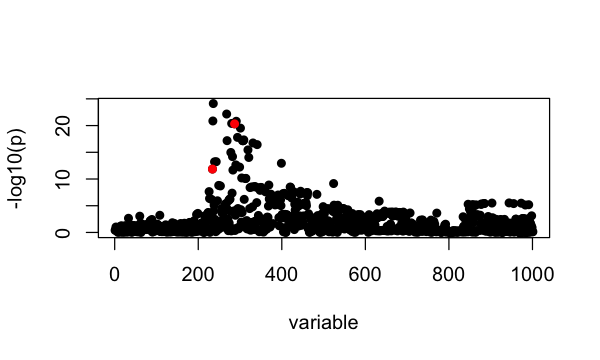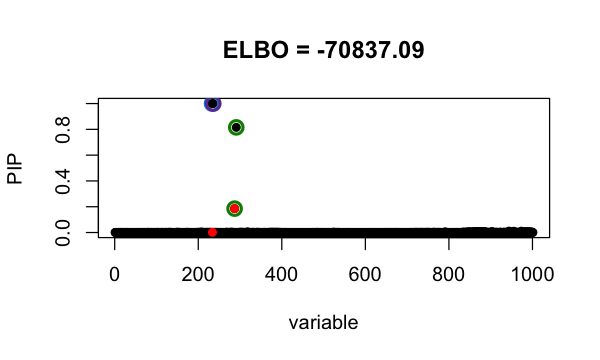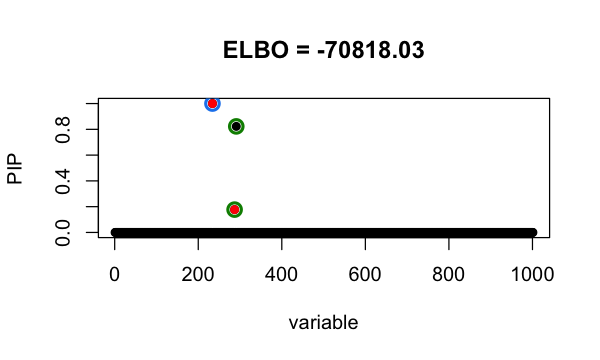# Refine SuSiE model

#### 2022-06-26

In this vignette, we demonstrate a procedure that helps SuSiE get out of local optimum.

We simulate phenotype using UK Biobank genotypes from 50,000 individuals. There are 1001 SNPs. It is simulated to have exactly 2 non-zero effects at 234, 287.

library(susieR)
library(curl)
data_file <- tempfile(fileext = ".RData")
data_url <- paste0("https://raw.githubusercontent.com/stephenslab/susieR/",
"master/inst/datafiles/FinemappingConvergence1k.RData")
b <- FinemappingConvergence$true_coef susie_plot(FinemappingConvergence$z, y = "z", b=b)The strongest marginal association is a non-effect SNP.

Since the sample size is large, we use sufficient statistics ($$X^\intercal X, X^\intercal y, y^\intercal y$$ and sample size $$n$$) to fit susie model. It identifies 2 Credible Sets, one of them is false positive. This is because susieR get stuck around a local minimum.

fitted <- with(FinemappingConvergence,
susie_suff_stat(XtX = XtX, Xty = Xty, yty = yty, n = n))
susie_plot(fitted, y="PIP", b=b, main=paste0("ELBO = ", round(susie_get_objective(fitted),2)))Our refine procedure to get out of local optimum is

1. fit a susie model, $$s$$ (suppose it has $$K$$ CSs).

2. for CS in $$s$$, set SNPs in CS to have prior weight 0, fit susie model –> we have K susie models: $$t_1, \cdots, t_K$$.

3. for each $$k = 1, \cdots, K$$, fit susie with initialization at $$t_k$$ ($$\alpha, \mu, \mu^2$$) –> $$s_k$$

4. if $$\max_k \text{elbo}(s_k) > \text{elbo}(s)$$, set $$s = s_{kmax}$$ where $$kmax = \arg_k \max \text{elbo}(s_k)$$ and go to step 2; if no, break.

We fit susie model with above procedure by setting refine = TRUE.

fitted_refine <- with(FinemappingConvergence,
susie_suff_stat(XtX = XtX, Xty = Xty, yty = yty,
n = n, refine=TRUE))
# WARNING: XtX is not symmetric; forcing XtX to be symmetric by replacing XtX with (XtX + t(XtX))/2
# WARNING: XtX is not symmetric; forcing XtX to be symmetric by replacing XtX with (XtX + t(XtX))/2
# WARNING: XtX is not symmetric; forcing XtX to be symmetric by replacing XtX with (XtX + t(XtX))/2
# WARNING: XtX is not symmetric; forcing XtX to be symmetric by replacing XtX with (XtX + t(XtX))/2
# WARNING: XtX is not symmetric; forcing XtX to be symmetric by replacing XtX with (XtX + t(XtX))/2
# WARNING: XtX is not symmetric; forcing XtX to be symmetric by replacing XtX with (XtX + t(XtX))/2
# WARNING: XtX is not symmetric; forcing XtX to be symmetric by replacing XtX with (XtX + t(XtX))/2
# WARNING: XtX is not symmetric; forcing XtX to be symmetric by replacing XtX with (XtX + t(XtX))/2
# WARNING: XtX is not symmetric; forcing XtX to be symmetric by replacing XtX with (XtX + t(XtX))/2
# WARNING: XtX is not symmetric; forcing XtX to be symmetric by replacing XtX with (XtX + t(XtX))/2
susie_plot(fitted_refine, y="PIP", b=b, main=paste0("ELBO = ", round(susie_get_objective(fitted_refine),2)))With the refine procedure, it identifies 2 CSs with the true signals, and the achieved evidence lower bound (ELBO) is higher.

## Session information

Here are some details about the computing environment, including the versions of R, and the R packages, used to generate these results.

sessionInfo()
# R version 3.6.2 (2019-12-12)
# Platform: x86_64-apple-darwin15.6.0 (64-bit)
# Running under: macOS Catalina 10.15.7
#
# Matrix products: default
# BLAS:   /Library/Frameworks/R.framework/Versions/3.6/Resources/lib/libRblas.0.dylib
# LAPACK: /Library/Frameworks/R.framework/Versions/3.6/Resources/lib/libRlapack.dylib
#
# locale:
#  en_US.UTF-8/en_US.UTF-8/en_US.UTF-8/C/en_US.UTF-8/en_US.UTF-8
#
# attached base packages:
#  stats     graphics  grDevices utils     datasets  methods   base
#
# other attached packages:
#  curl_4.3       Matrix_1.2-18  L0Learn_1.2.0  susieR_0.12.16
#
# loaded via a namespace (and not attached):
#   tidyselect_1.1.1   xfun_0.29          bslib_0.3.1        reshape2_1.4.3
#   purrr_0.3.4        lattice_0.20-38    colorspace_1.4-1   vctrs_0.3.8
#   generics_0.0.2     htmltools_0.5.2    yaml_2.2.0         utf8_1.1.4
#  rlang_0.4.11       mixsqp_0.3-46      jquerylib_0.1.4    pillar_1.6.2
#  glue_1.4.2         DBI_1.1.0          RcppZiggurat_0.1.5 matrixStats_0.61.0
#  lifecycle_1.0.0    plyr_1.8.5         stringr_1.4.0      munsell_0.5.0
#  gtable_0.3.0       evaluate_0.14      knitr_1.37         fastmap_1.1.0
#  irlba_2.3.3        parallel_3.6.2     fansi_0.4.0        Rfast_2.0.3
#  highr_0.8          Rcpp_1.0.7         scales_1.1.0       jsonlite_1.7.2
#  ggplot2_3.3.5      digest_0.6.23      stringi_1.4.3      dplyr_1.0.7
#  grid_3.6.2         tools_3.6.2        magrittr_2.0.1     sass_0.4.0
#  tibble_3.1.3       crayon_1.4.1       pkgconfig_2.0.3    ellipsis_0.3.2
#  assertthat_0.2.1   rmarkdown_2.11     reshape_0.8.8      R6_2.4.1
#  compiler_3.6.2